# Elliptic Function: JF

For rectangular tori the Jacobi sn Function and the jf-function differ only by a real scaling factor. Both have their 4 branch values (i.e. where the derivative vanishes) on the real axis and symmetric to the imaginary axis.

The sn-function has one pair of branch values on the unit circle, the jf-function has its branch values symmetric to the unit circle. The cross ratio of the branch values is determined by the torus.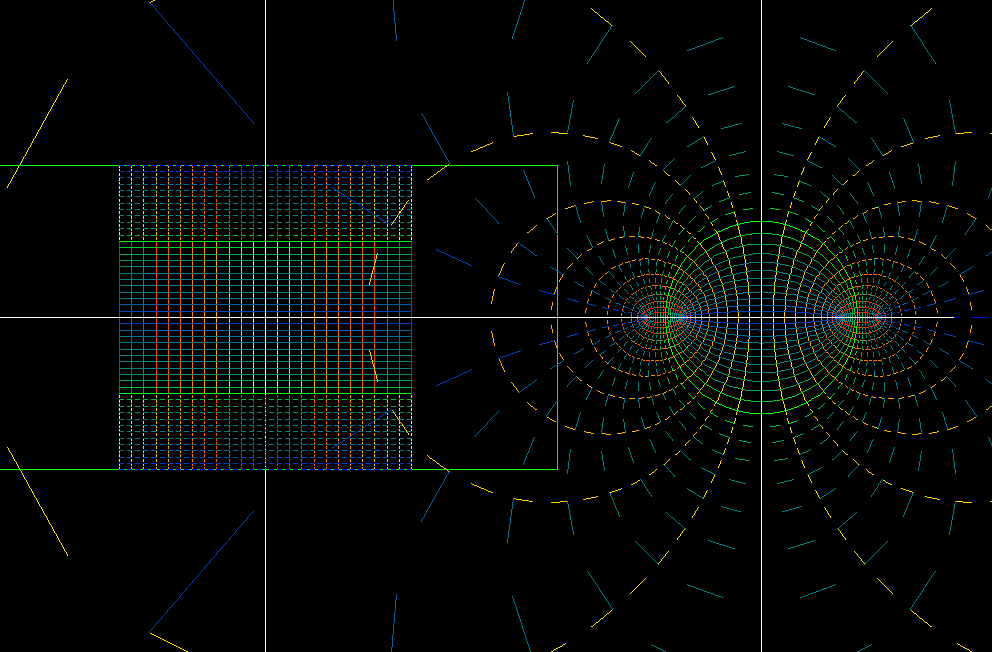domain and range of 10 doubly periodic elliptic functions. Their fundamental domains are centered at the origin. The grid part in the domain is mapped to the whole sphere, and the non-grid half also.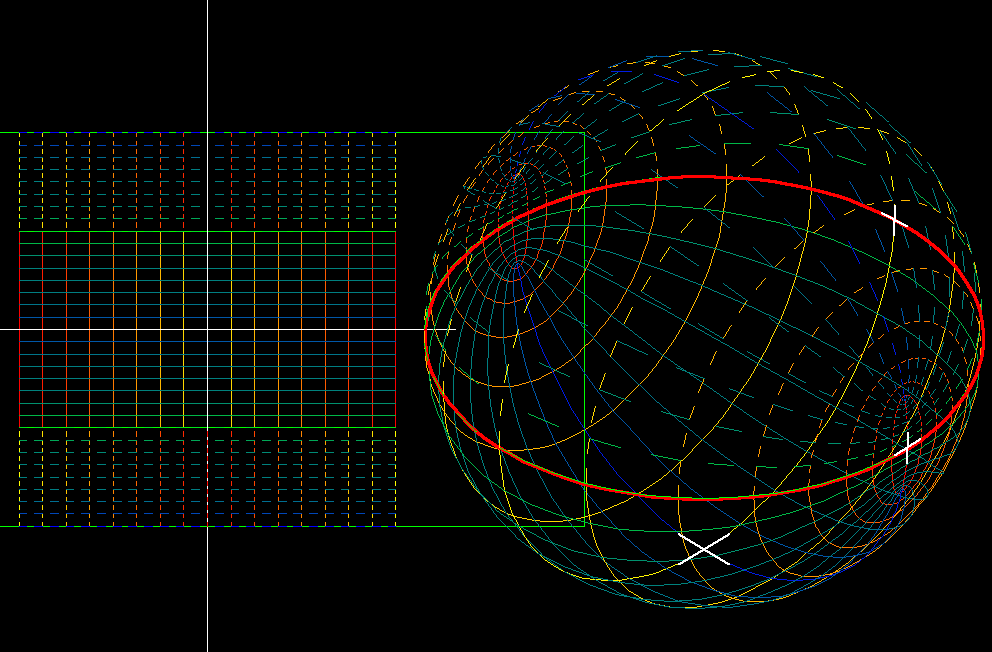The values of the 10 doubly periodic functions with rectangular fundamental domain (for the translation symmetries) are shown on the Riemann sphere. Observe the symmetric position of the four branch values.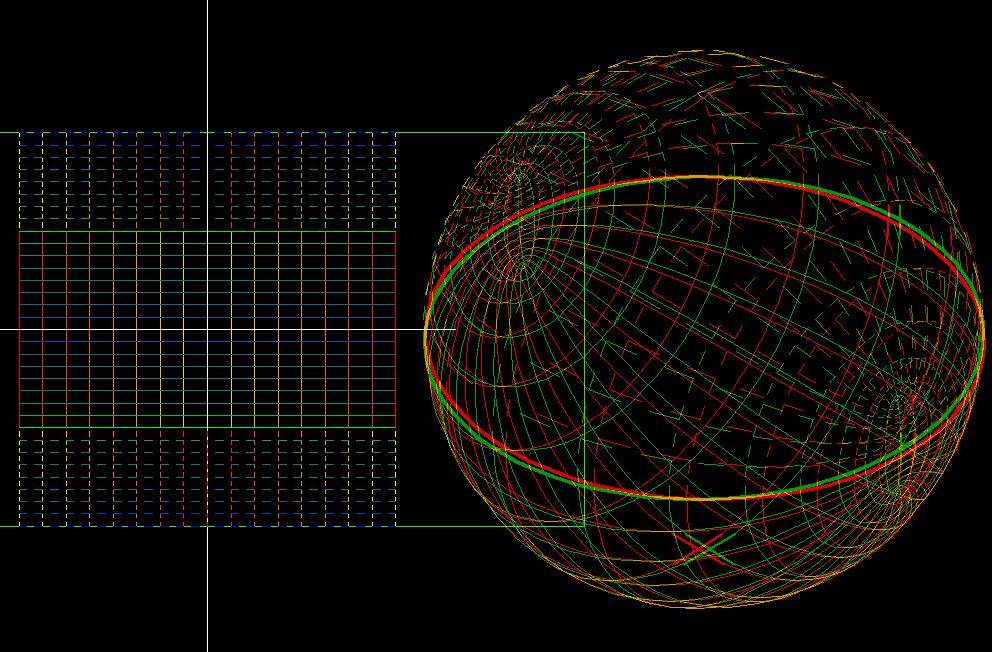doubly periodic functions with rectangular fundamental domains. Values on the Riemann sphere, anaglyph.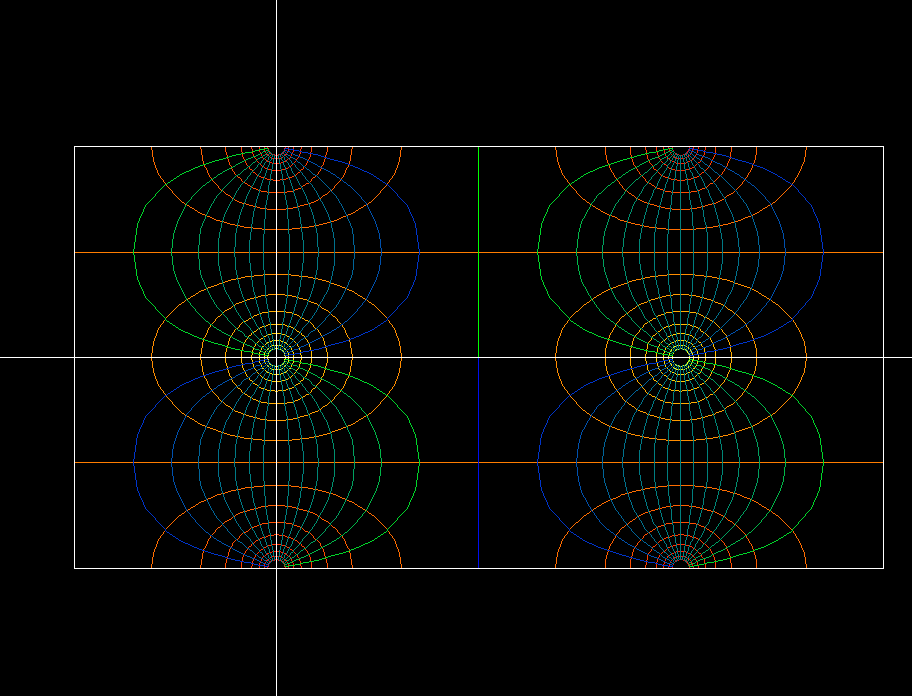Inverse image of a conformal polar grid on the Riemann sphere under the doubly periodic functions shown above. The left half of the fundamental domain is mapped so that it covers the sphere and the same for the right half. The polar centers are the preimages of zero and infinity. The two preimages of zero halve a branch point in the middle between them (the same for the two preimages of infinity). The boundaries of the subrectangles are preimages of the real axis. The insides are the preimages of the upper and the lower half plane.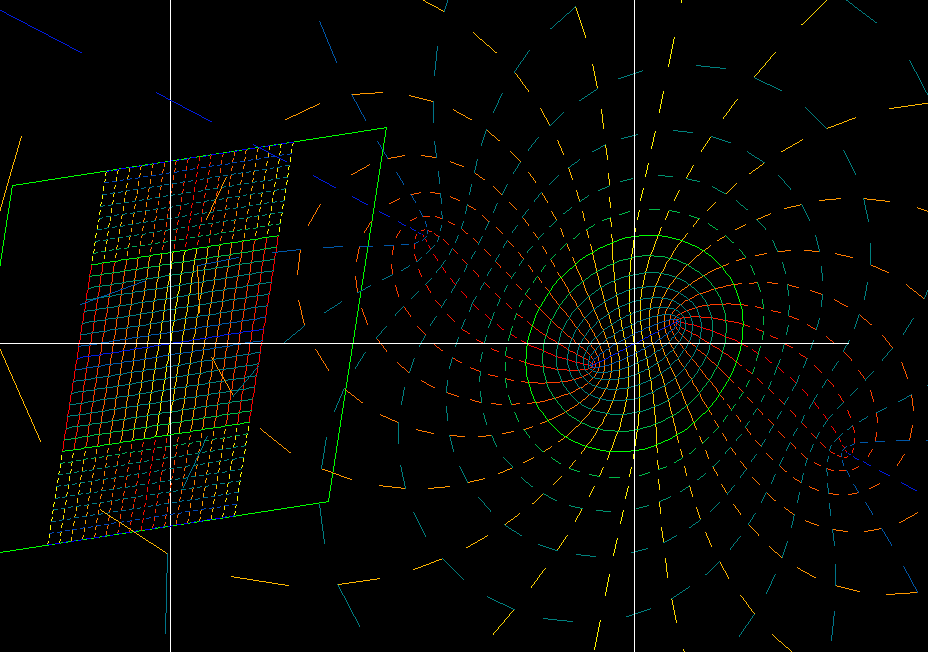10 doubly periodic “elliptic” functions with rhombic fundamental domains. The gridded middle half of the rhombic domain is mapped to the whole sphere, and the other half also covers the sphere.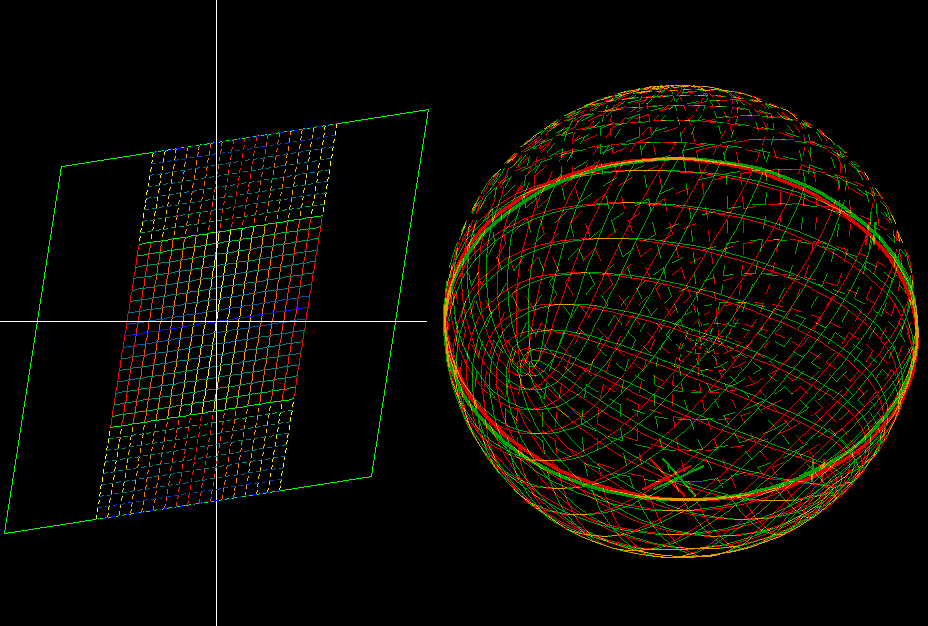11 doubly periodic functions with rhombic fundamental domains. Their values are shown on the Riemann sphere, anaglyph drawing. If B is one branch value, then -B, 1/B, -1/B are the others.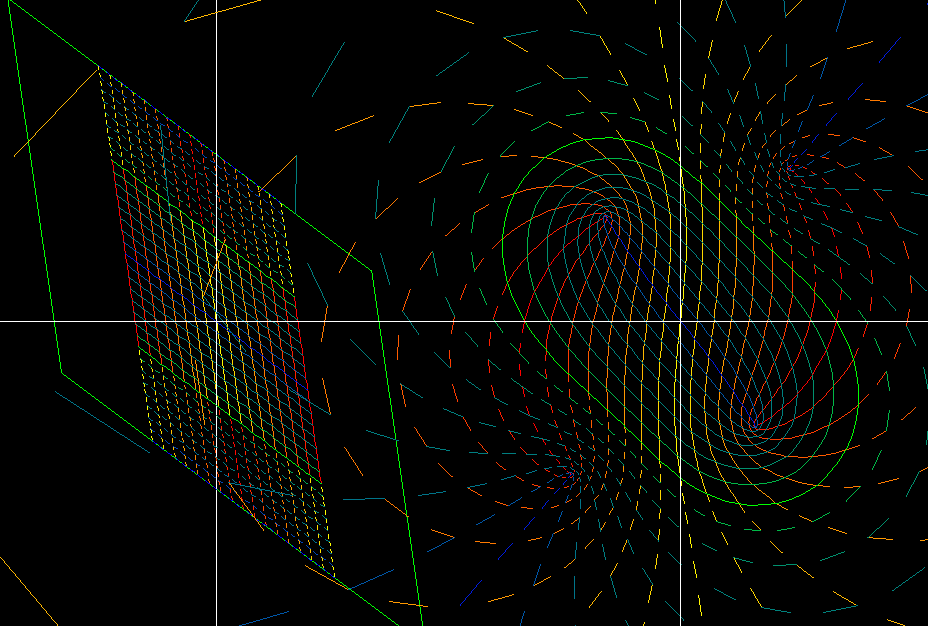11 doubly periodic functions with parallelogram fundamental domains (for the translation symmetries). Again, the gridded middle half of the fundamental domain is mapped to the full Riemann sphere (or Gaussian plane), and the not gridded half also.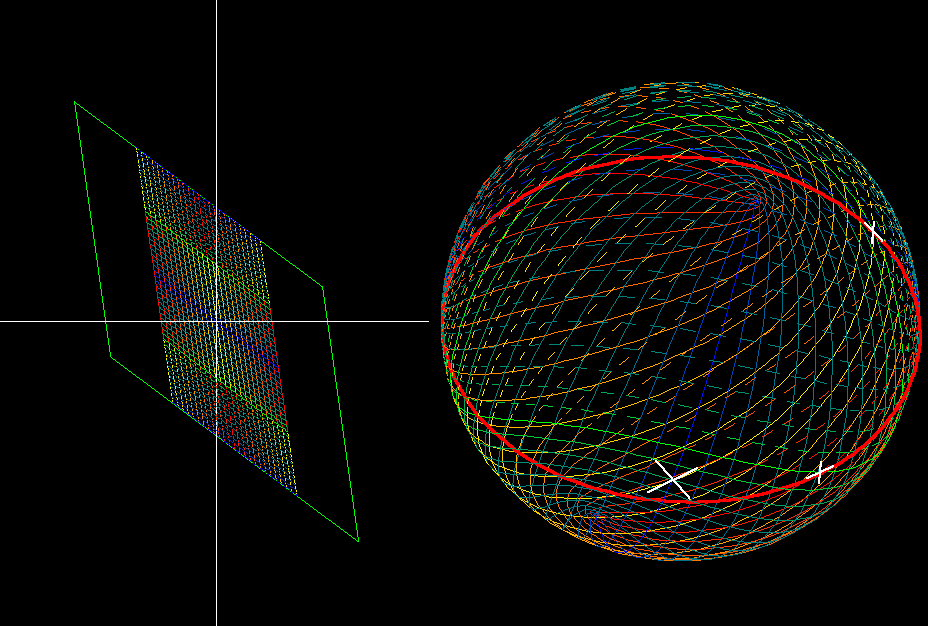The same 11 doubly periodic functions, their values shown on the Riemann sphere.

z_elliptic_functions.pdf